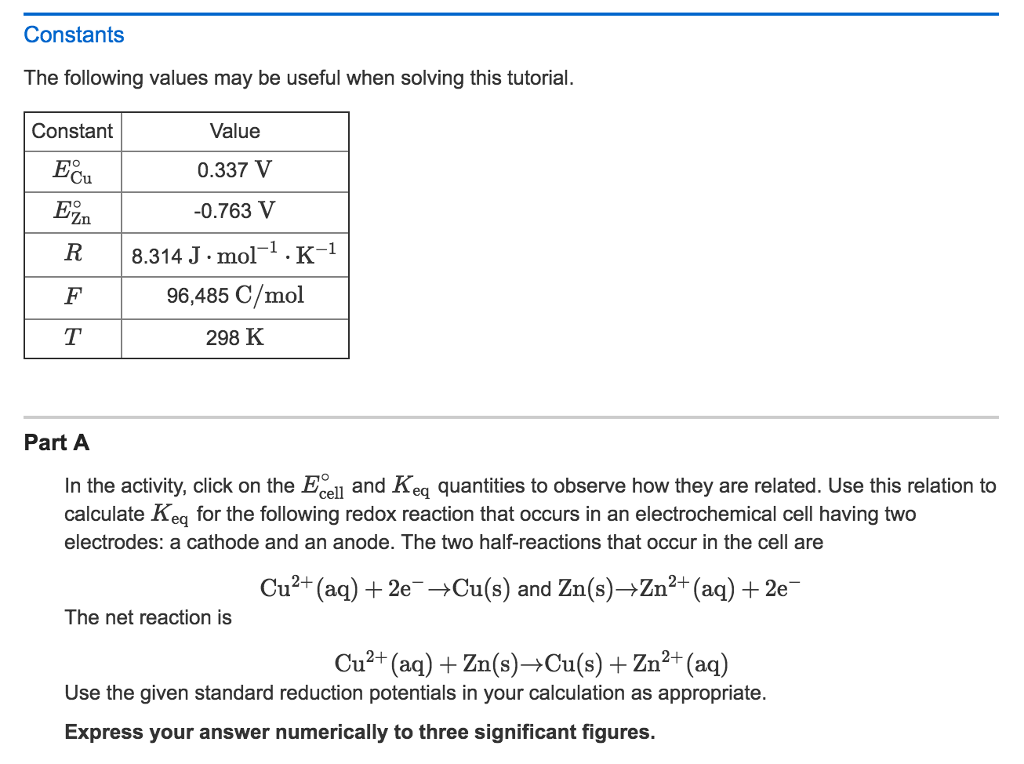# Question & Answer: Constants The following values may be useful when solving this tutorial. Part A In the activity,…..Constants The following values may be useful when solving this tutorial. Part A In the activity, click on the E degree _cell and K_eq quantities to observe how they are related. Use this relation to calculate K_eq for the following redox reaction that occurs in an electrochemical cell having two electrodes: a cathode and an anode. The two half-reactions that occur in the cell are Cu^2+ (aq) + 2e^- rightarrow Cu(s) and Zn(s) rightarrow Zn^2+ (aq) + 2e^- The net reaction is Cu^2+ (aq) + Zn(s) rightarrow Cu(s) + Zn^2+ (aq) Use the given standard reduction potentials in your calculation as appropriate. Express your answer numerically to three significant figures.

First, relate Eºcell

Don't use plagiarized sources. Get Your Custom Essay on
Question & Answer: Constants The following values may be useful when solving this tutorial. Part A In the activity,…..
GET AN ESSAY WRITTEN FOR YOU FROM AS LOW AS \$13/PAGE

dG = -RT*ln(Keq)

dG = -n*F*Eºcell

therefore

n*F*Eºcell = RT*ln(Keq)

substitute data

Eºcell = Ered – Eox = 0.337 – – 0.763 = 1.1 V

n*F*Eºcell = RT*ln(Keq)

2*96500*1.1 = 8.314*298*ln(Keq)

lnKeq = 2*96500*1.1/(8.314*298)

Keq = exp(85.6887)

Keq = 1.6373*10^37

Keq = 1.64*10^37 (to 3 sig. fig)